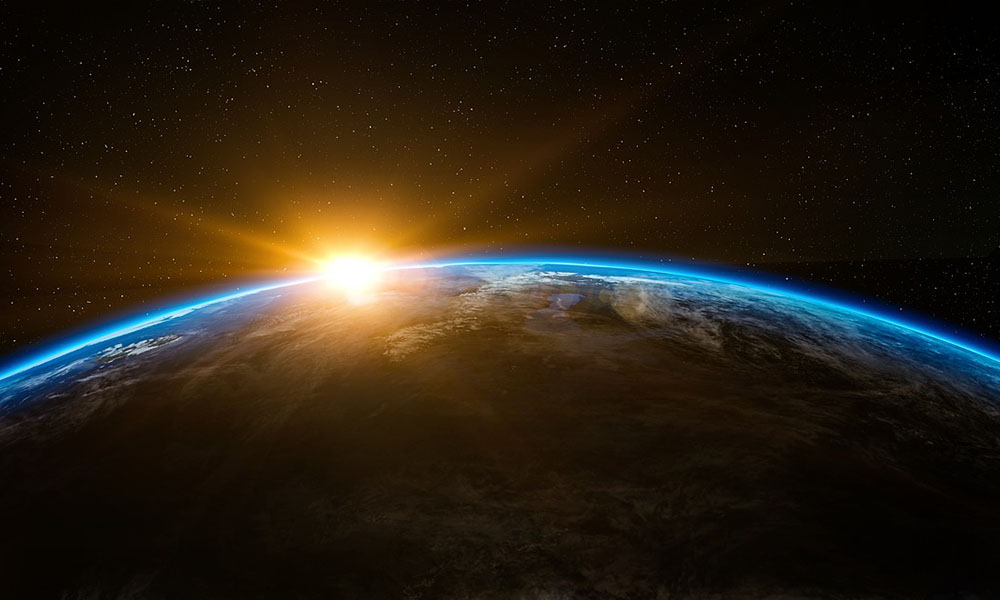# The Thickness of Earth’s Crust

If Earth has expanded to a diameter twice that of its original size, and this has happened with no addition of matter, as proposed by Peter Woodhead, we can calculate that the crust of our planet is no more than 287 km thick. The math for this can be found at the end of this post.

This does not square well with Jan Lamprecht’s observation that the deepest earthquakes we come across have their epicenter about 700 km below ground, which suggest to us that Earth’s crust is at least 700 km thick.

Earth’s expansion must in part have been due to added matter. The mechanism for this, suggested in my book, is radioactive decay. When atoms decay, they multiply. Where there once was one atom, there are suddenly two. The volume of matter increase when it decays through radiation.

For the crust to be 700 km, rather than 287 km thick, the bulk of our planet’s crust must have more than doubled from when it was created, assuming a doubling of its radius over this same time period.Cross section of a hollow planet with internal electric pressure

Here’s the calculation for Earth’s crust, assuming no additional matter, as proposed by Peter Woodhead:

The formula for the volume of a sphere is V = 4/3 pi r^3

Let volume of Earth’s current crust be V_crust
Let volume of Earth’s current hollow be V_hollow
Let V_total = V_crust + V_hollow
Let volume of Earth’s original crust be V_original
Let volume of Earth’s original hollow be 0

Since Earth expanded with no added matter, we have V_crust = V_original

From above, we have V_crust = V_total – V_hollow

Substitute V_original = V_total – V_hollow

Solve for V_hollow we get V_hollow = V_total – V_original

4/3 pi r_hollow^3 = 4/3 pi r_total^3 – 4/3 pi r_original^3

Simplify by getting rid of constants: r_hollow^3 = r_total^3 – r_original^3

Let r_total be 2 and r_original be 1, to reflect the idea that r_original is half of r_total:

r_hollow^3 = 8 – 1
r_hollow = 7^(1/3)

r_hollow = 1.91
r_total = 2
r_original = 1

From this we get that the radius of the hollow is 95.5 % of the total current radius. Only 4.5% of the radius is the crust. Given that Earth’s radius is 6371 km, we get that its crust is currently 287 km thick.

#### This Post Has 0 Comments

This site uses Akismet to reduce spam. Learn how your comment data is processed.